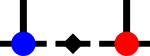SyTen## ◆ prod_impl() [1/2]

template<Rank summed, Rank frank, Rank srank>
 std::enable_if_t > syten::TensorProd::prod_impl ( SourceLocation location, Tensor< frank > const & a, Tensor< srank > const & b, std::array< int, frank > const & c_a, std::array< int, srank > const & c_b, Conj const conj = Conj::n(), EliminateZeros const ezeros = EliminateZeros::No, HandleFermions const handle_ferms = HandleFermions::n(), ConstSpan< Index > ferm_a = ConstSpan(), ConstSpan< Index > ferm_b = ConstSpan(), ConstSpan< Bool > parity_a = ConstSpan(), ConstSpan< Bool > parity_b = ConstSpan() )
inline

Implementation of the tensor-tensor-to-tensor product.

This implementation takes care to both minimise memory usage and at the same time keep the order of tensor blocks as handed down from the ancients (aka ‘which just happens to work’).

Parameters
 location Callsite identifier. a first tensor to be contracted b second tensor to be contracted c_a contraction spec for a, legs with positive indices here are contracted c_b contraction spec for b, legs with positive indices here are contracted conj if this is Conj::y(), b is complex-conjugated ezeros if this is EliminateZeros::Yes, close-to-zero entries are set to zero handle_ferms if this is true, fermionic commutation is handled in a bare-bones manner ferm_a effective fermionic order of a tensor, initialised only if handle_ferms is yes ferm_b effective fermionic order of b tensor, initialised only if handle_ferms is yes parity_a list of booleans specifying if additional parities are to be placed on a-tensor legs, initialised only if handle_ferms is yes parity_b list of booleans specifying if additional parities are to be placed on b-tensor legs, initialised only if handle_ferms is yes
Remarks
handle_ferms only takes care of minus signs from permutations of a and b to go from ferm_a and ferm_b to a fermionic order in which the contraction itself can happen without additional minus signs. It assumes that in the result tensor, legs of B come first in descending order (-2 first, then -4, then -5) and legs of A come second in descending order (-1 first, then -3). It also assumes that ferm_b matches the effective order of b taking into account conj. That is, when calling the method with conj = Conj::y(), you also need to reverse ferm_b.

Get the number of component labels required per tensor leg.

For every bucket in a, find the matching bucket in b.

See issue K9ABw

Now sort the proto-blocks of protolist into bins according to their symmetry sectors. Each bin also gets an integer of the "completed" blocks and a mutex protecting that integer. If the number of completed blocks is equal to the number of elements in the bin, the last thread reduces the bin.Here is the call graph for this function: